# Introduction to tidy spatial networks

Written and presented by Stéphane Guillou Technology Trainer, UQ Library Mastodon: @stragu@mastodon.indie.host

This tutorial is an introduction to dealing with tidy spatial networks in R, demonstrating a full process of data acquisition from the open spatial database OpenStreetMap, data preparation, and basic network analysis like isodistance and shortest path calculation. Along the way, we use the default plotting methods for spatial network objects, but also make use of the ggplot2 and tmap packages as alternatives.

## Setting up

To follow this tutorial, you will have to have a number of packages available, which can be best sorted out with the following command:

``install.packages(c("dplyr", "ggplot2", "sfnetworks", "tmap", "osmdata"))``

If you run into issues installing and running sf (which relies on spatial libraries external to R), please refer to their installation instructions.

## sfnetworks

The main package this tutorial centres around is the sfnetworks package (Lucas van der Meer, Lorena Abad et al.), which is the result of joining what the tidygraph package does for networks with what the sf package does for spatial vector data.

The data structure central to this package is a combination of two spatial objects, one describing the nodes (or points) and another describing the edges (or lines connecting the points). In the words of the developers:

“A close approximation of tidyness for relational geospatial data is two sf objects, one describing the node data and one describing the edge data.”

In other words, an `sfnetwork` object is made up of one `sf` object for nodes and one `sf` object for edges. (As opposed to two simple `data.frame`s for a tidygraph object.)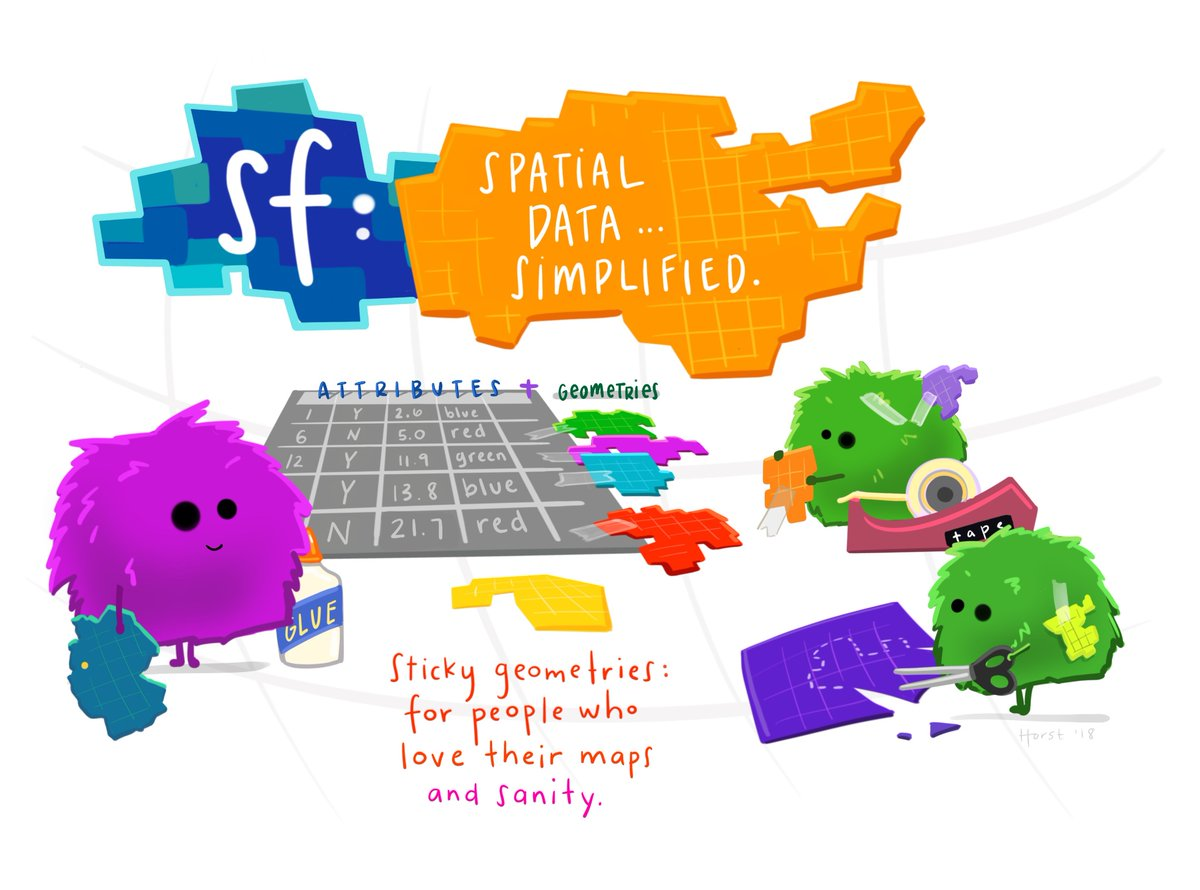A visual explanation of sf objects by Allison Horst (copyright hers): a dataframe with sticky geometries in a list column. The geometries are “sticky” because they don’t vanish when, say, you `select()` columns.

In the edges component of a network, it is also possible (or necessary) to specify where the edge starts and finishes, using the `from` and `to` columns. Such information can be interpreted as the direction of the edge in a “directed” network, or simply as its two extremities in the case of an “undirected” network.

Finally, another important characteristic of the edges is their weight. This can relate to any kind of information, but if we are interested in distances between points, it will typically be the length of the edges.

## From OSM data to an sfnetwork

Although the structure of an `sfnetwork` seems to be quite complex, it is possible – and useful! – to use a shortcut and create one from a simple collection of linestrings.

We can download download data from a spatial vector database like OpenStreetMap (OSM), focus on the lines (or “ways”) and convert that `sf` object into an `sfnetwork` that will use the lines as edges, and their endpoints as nodes.

Let’s first download from OSM all the ways that are primary used by pedestrians in the suburb of West End. (To understand how these features are tagged in the database, a good starting point is the Map Features page on the OSM wiki.)

``library(osmdata)``
``````# build the query
we_foot <- opq("west end, meanjin") %>%
'"highway"="steps"',
'"foot"="yes"',
'"highway"="living_street"')) %>%
osmdata_sf()``````

The osmdata package allows building Overpass queries to download OSM vector data that matches our criteria:

• Features tagged as `highway=footway`, including footpaths, crossings…
• Features tagged as `highway=steps`, for stairs
• Features tagged as `foot=yes`, for example bikeways on which foot traffic is allowed (see this shared bikeway for an example)
• Features tagged as `highway=living_street`, a shared road on which pedestrians often have right of way

Finding the right combination of OSM tags to use is always an iterative process, refining the selection at each step.

We choose to return an `sf` object, which might contain points, lines and polygons. We are only interested in the lines:

``names(we_foot)``
``````##  "bbox"              "overpass_call"     "meta"
##  "osm_points"        "osm_lines"         "osm_polygons"
##  "osm_multilines"    "osm_multipolygons"``````
``we_foot_lines <- we_foot\$osm_lines``

We can visualise the resulting object with the sf package:

``````library(sf)
we_foot_lines %>%
st_geometry() %>%
plot()``````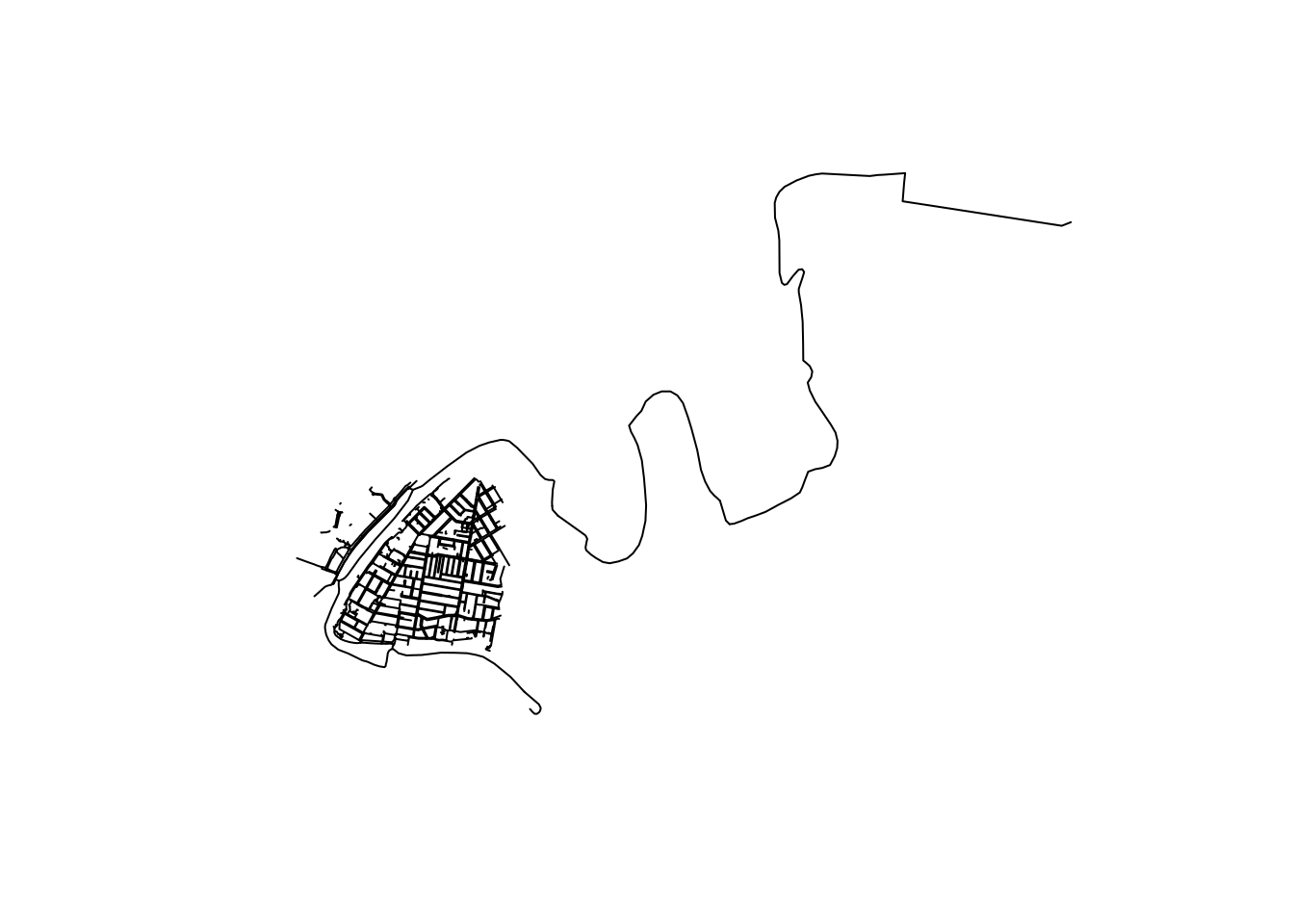It seems to have kept a ferry route, which was tagged with `foot=yes` and `route=ferry` on OSM. We can remove it:

``````library(dplyr)
we_foot_lines <- we_foot_lines %>%
filter(is.na(route))``````

Another caveat in our process is that some footpaths are mapped as closed ways on OSM, and are therefore returned as a polygon:

``````we_foot\$osm_polygons %>%
st_geometry() %>%
plot()``````The smaller ones are proper pedestrian areas, but the bigger ones are probably footpaths encircling a whole residential block. It is possible to “cast” these polygons as ways and include them in the network:

``````# cast polygons to lines
poly_to_lines <- st_cast(we_foot\$osm_polygons, "LINESTRING")
# bind all lines together
library(dplyr)
we_foot_lines <- bind_rows(we_foot_lines, poly_to_lines)``````

This is what we are left with:

``````# plot it
we_foot_lines %>%
st_geometry() %>%
plot()``````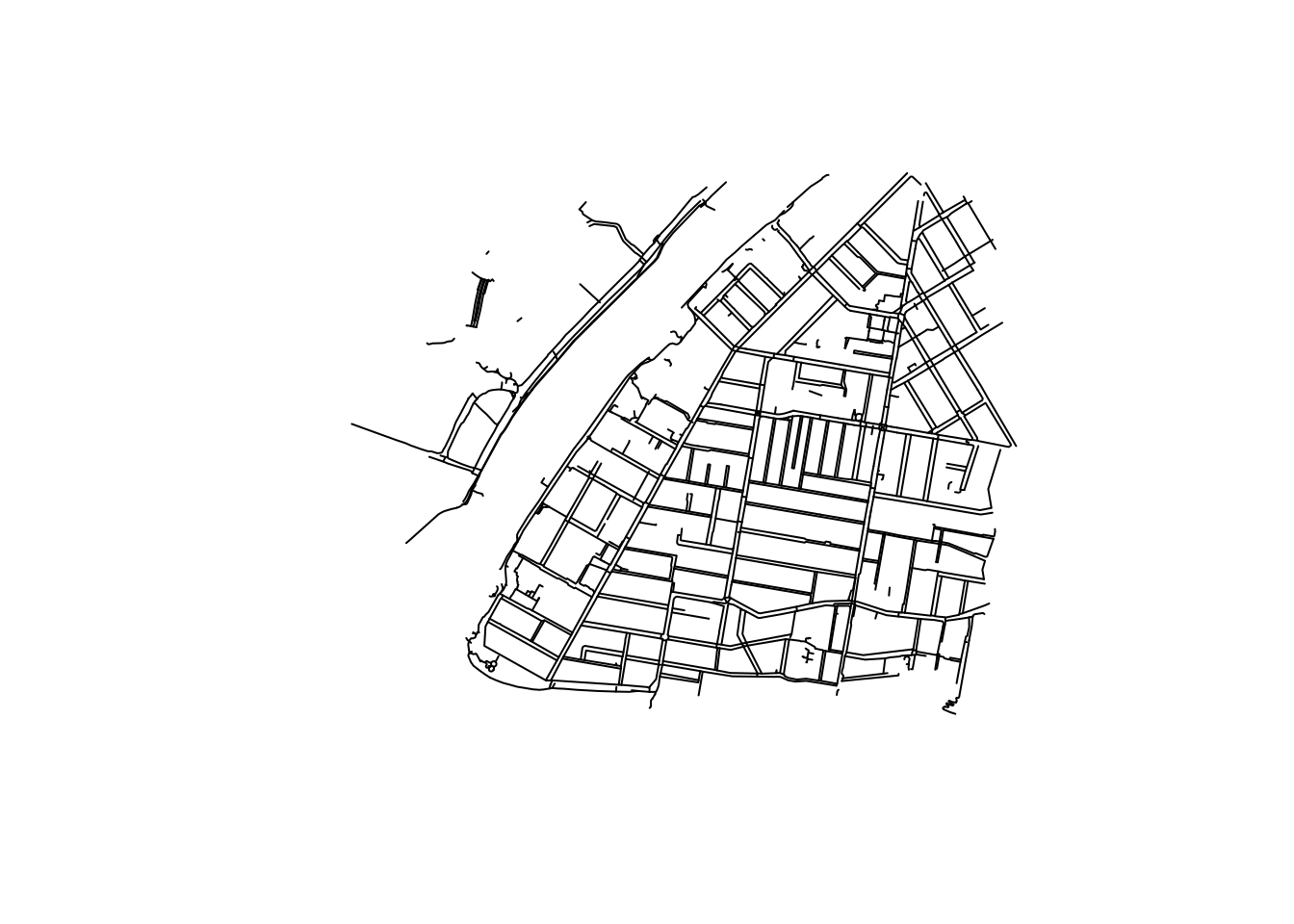We can now convert the object to an `sfnetwork` object, making sure we set it as undirected (the default is `directed = TRUE`):

``````library(sfnetworks)
foot_net <- as_sfnetwork(we_foot_lines, directed = FALSE)
plot(foot_net)``````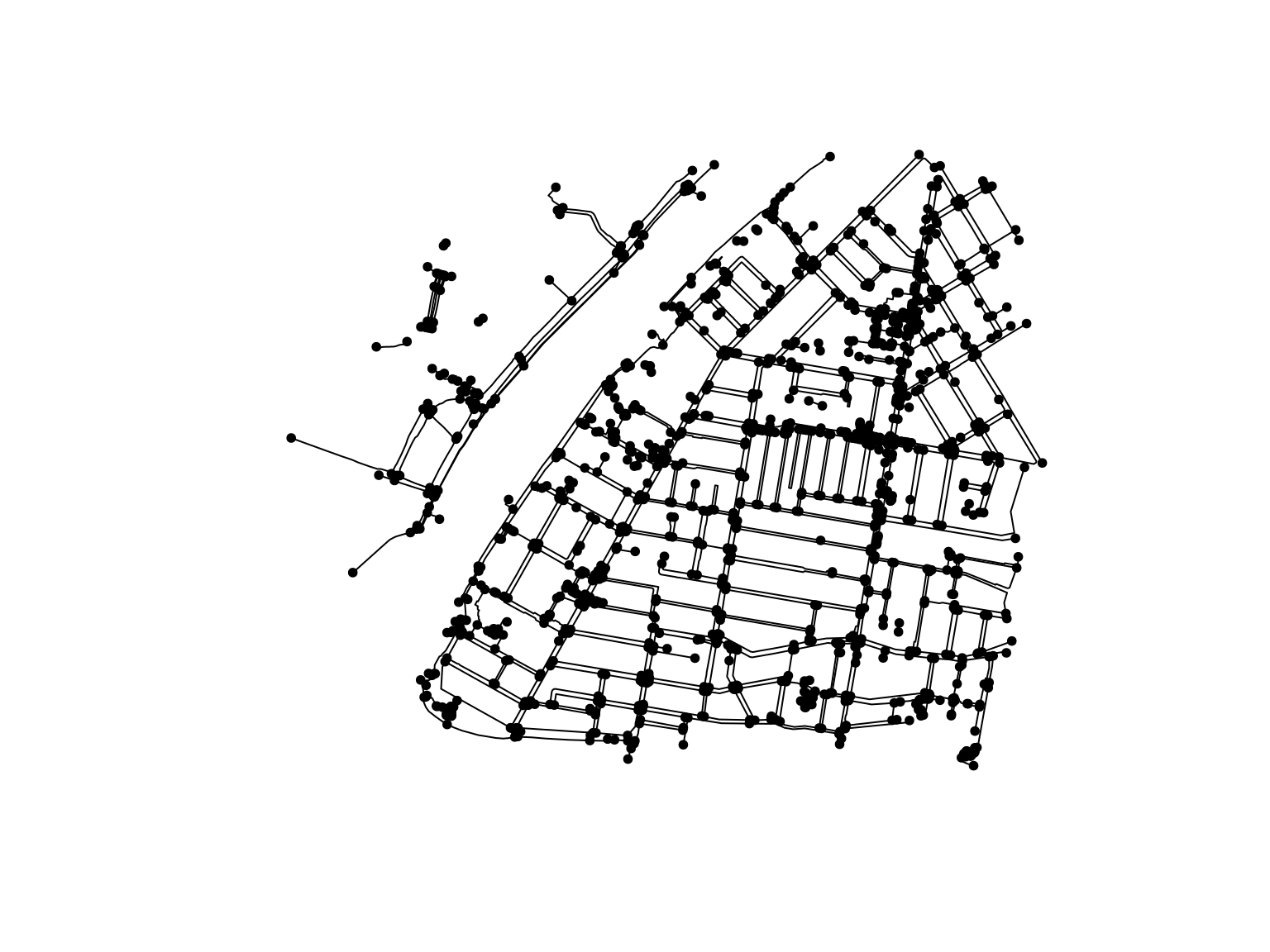## Prepare the network

### CRS

The current coordinate system for the network is a global one, EPSG:4326.

``st_crs(foot_net)``
``````## Coordinate Reference System:
##   User input: EPSG:4326
##   wkt:
## GEOGCRS["WGS 84",
##     ENSEMBLE["World Geodetic System 1984 ensemble",
##         MEMBER["World Geodetic System 1984 (Transit)"],
##         MEMBER["World Geodetic System 1984 (G730)"],
##         MEMBER["World Geodetic System 1984 (G873)"],
##         MEMBER["World Geodetic System 1984 (G1150)"],
##         MEMBER["World Geodetic System 1984 (G1674)"],
##         MEMBER["World Geodetic System 1984 (G1762)"],
##         ELLIPSOID["WGS 84",6378137,298.257223563,
##             LENGTHUNIT["metre",1]],
##         ENSEMBLEACCURACY[2.0]],
##     PRIMEM["Greenwich",0,
##         ANGLEUNIT["degree",0.0174532925199433]],
##     CS[ellipsoidal,2],
##         AXIS["geodetic latitude (Lat)",north,
##             ORDER,
##             ANGLEUNIT["degree",0.0174532925199433]],
##         AXIS["geodetic longitude (Lon)",east,
##             ORDER,
##             ANGLEUNIT["degree",0.0174532925199433]],
##     USAGE[
##         SCOPE["Horizontal component of 3D system."],
##         AREA["World."],
##         BBOX[-90,-180,90,180]],
##     ID["EPSG",4326]]``````

`st_transform()` allows us to transform the coordinates to a different projection. For this part of the world, a recent Projected Reference System is EPSG:7856 (or “GDA2020 / MGA zone 56”).

``foot_net <- st_transform(foot_net, 7856)``

If the command above gives you a GDAL error, reassign the original CRS first: `st_crs(foot_net) <- 4326`

### Clean up

sfnetworks and tidygraph include many pre-processing and cleaning functions for graphs, some of them detailed in this article.

One relevant example for this dataset is the subdivision of edges: because some edges have interior nodes that are endpoints of other edges, they will not be connected to each other when analysing the network.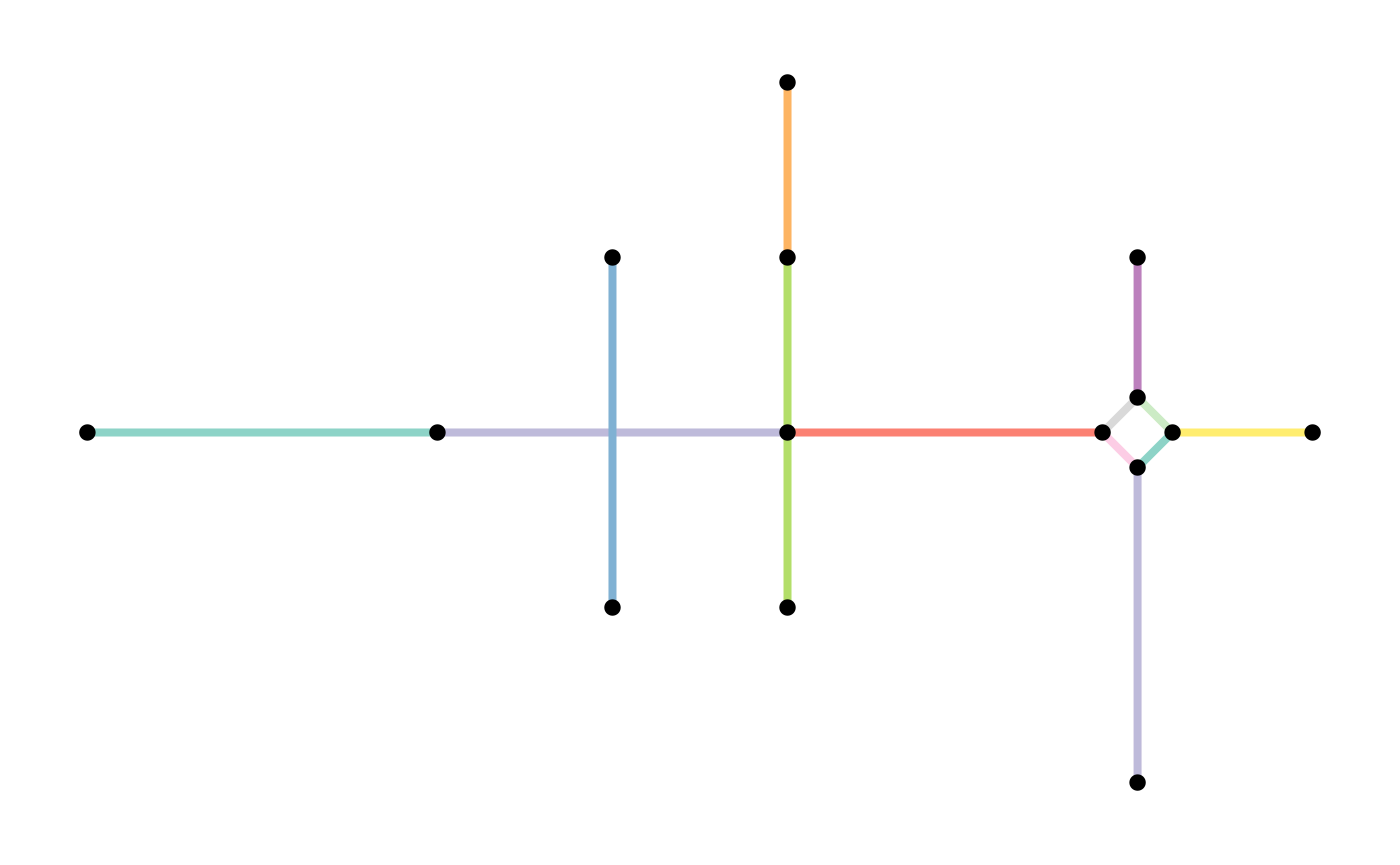We need to combine tidygraph’s `convert()` function with a “spatial morpher” function from sfnetworks:

``````library(tidygraph)
foot_net <- convert(foot_net, to_spatial_subdivision)``````

The network should now contain more edges.

Note that this will only happen for features sharing a node: if two edges overlap, like for bridges and underpaths, no extra node will be created where they cross, and therefore no new connecting endpoints will be created for them – which is good news!

Also note that using `to_spatial_subdivision()` will copy tags into each of the subdivisions of an edge. This usually isn’t a problem (think speed limits, surface, lighting, width…) but can be in some cases (for example if the length of the edge had already been added). The order of processing often matters.

Another example is the removal of nodes that have only two edges connected, also called “smoothing of pseudo-nodes”. This would be useful to simplify a network and reduce the processing times needed. We again use a combination of `convert()` with the relevant spatial morpher, `to_spatial_smooth()`:

``````foot_simple <- convert(foot_net, to_spatial_smooth)
plot(foot_simple)``````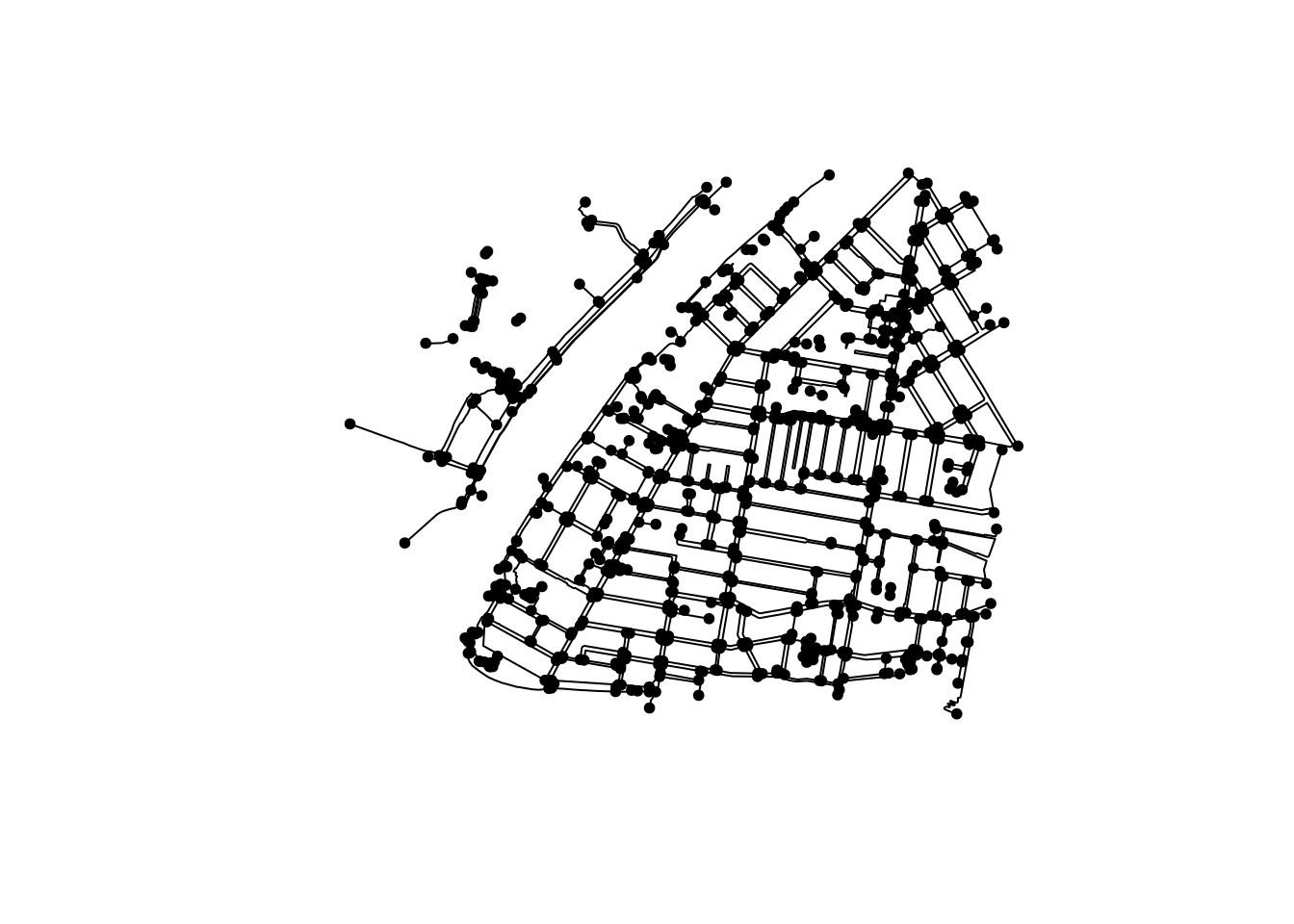For our example, simplifying the network might not be useful:

• If we calculate isodistances or isochrones, reducing the number of nodes will reduce the precision;
• Once again, one needs to take care of the potential loss of valuable data. For example, do nodes contain relevant tags, like ones describing physical barriers? And how will the combined edges’ tags be stored?

More information about “spatial morphers”, including what options exist for dealing with attributes when multiple features are merged, is available in the documentation: `?spatial_morphers`

### Weights

As mentioned before, a common weight associated with each edge of the network is the edge’s length. We can add this weight to our network, but we need to first “activate” the part of the object we want to modify:

``````foot_net <- foot_net %>%
activate("edges") %>%
mutate(weight = edge_length())``````

Using `st_as_sf()`, we can extract the components of an `sfnetwork` object and use them in a familiar plotting system like ggplot2:

``````library(ggplot2)
ggplot() +
geom_sf(data = st_as_sf(foot_net, "edges"),
mapping = aes(colour = as.numeric(weight))) +
labs(colour = "Edge length (m)")``````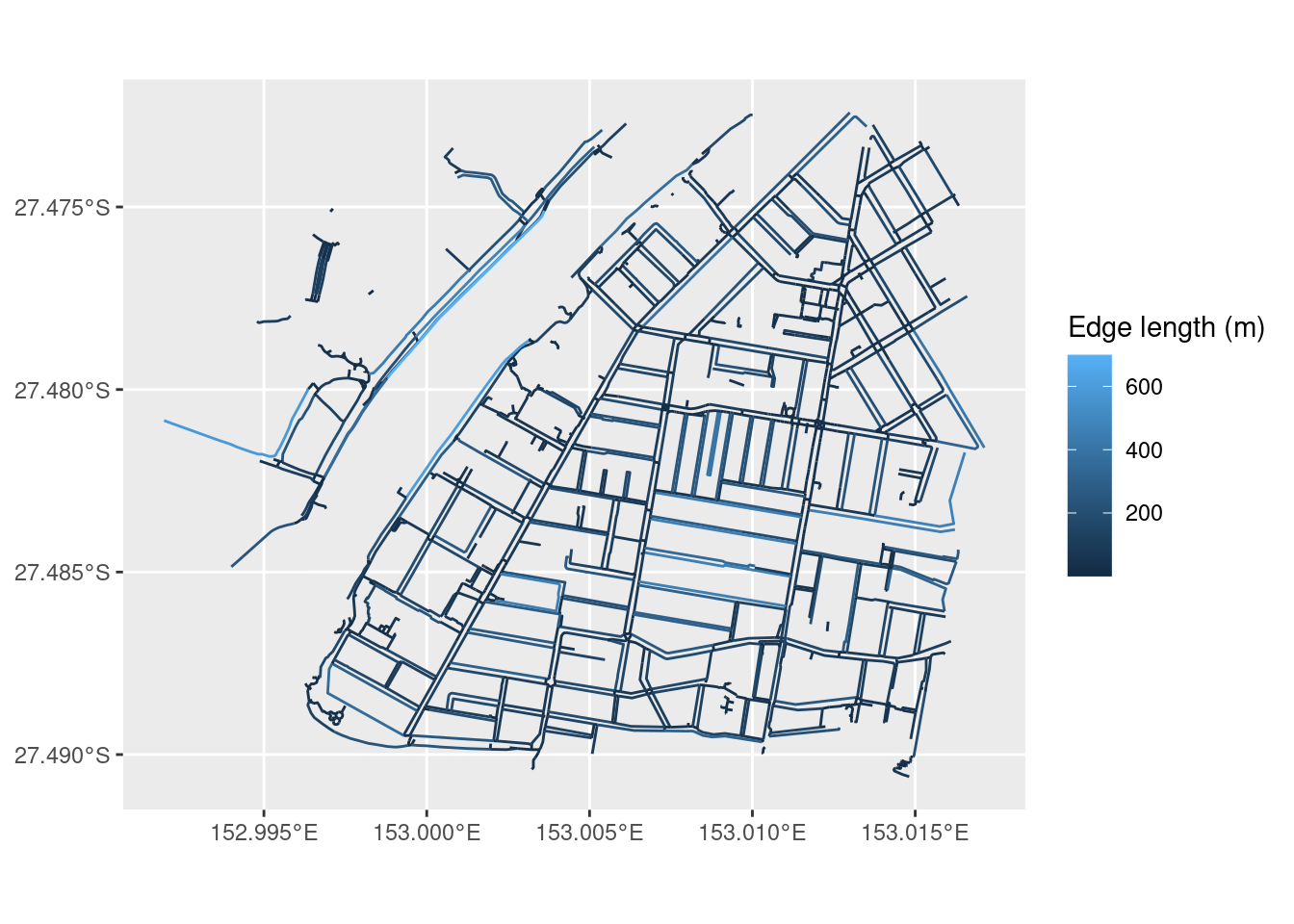## Interactive map

At this point, it might be interesting to create an interactive visualisation to zoom into, which might help spot issues with the data.

``````library(tmap)
tmap_mode("view") # set to interactive mode
tm_tiles("CartoDB.Positron") +
tm_shape(st_as_sf(foot_net, "edges")) +
tm_lines(col = "footway", palette = "Accent", colorNA = "red") +
tm_shape(st_as_sf(foot_net, "nodes")) +
tm_dots()``````